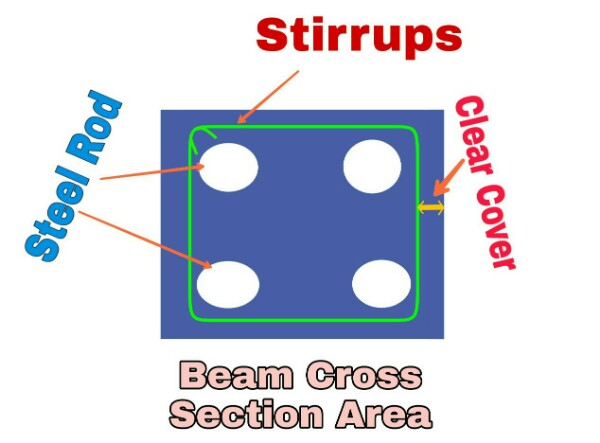# Percentage (%) of Rebar in Beam/Column/Slab Calculator

## Percentage of Rebar in Beam/Column/Slab Calculator.......................................................

.......................................................

## Steps to calculate percentage (%) of rebar in beam, column and slab?

This calculator helps to calculate the percentage of rebar in the structural member like, column beam and slab. The member of the house must be strong enough to beam compressive and tensile force.

Appropriate percentage of rebar in column, beam and slab is important for a structure to be safe as well as economical.

So, here i have provided simple steps to use the above calculator to calculate the percentage of rebar in column, beam and slab.

Step 1:- Input all the dimension and rebar details in the structure like cross sectional length, width, thickness etc for beam, column and slab.

Step 2 :- Input the diameter and quantity of rebar provided in the cross section of the beam, column and slab.

Step 3:- Click on calculate button to get the result. You will get the actual cross sectional area of the structure, area of rebar provided and total percentage of rebar in the column beam and slab.

Step 4:- Have a great day!!

## How to calculate percentage of rebar in column?

Q) The cross-sectional dimension of column be 400 mm x 400 mm. and total quantity of 16 mm rebar be 6 and total quantity of 20 mm rebar is 4 in number. What is the percentage of rebar?

Solution:-

Given, length of column = 400 mm

Width of Column = 400 mm

Quantity of 16 mm rebar = 6 Nos.

Quantity of 20 mm rebar = 4 Nos.

Here,

Step 1:- Cross sectional area column

Ac = 400 x 400 = 160000 mm square.

Step 2:- Cross sectional area 16 mm of rebar

Ar16 = 3.14 x 8 x 8 x 6 = 1205.76 mm square

(Since, 3.14 is value of pie. and 8 is the radius of rebar and 6 is quantity of rebar)

Step 3:- Cross sectional area 20 mm of rebar

Ar20 = 3.14 x 10 x 10 x 4 = 1256 mm square

(Since, 3.14 is value of pie. and 10 is the radius of rebar and 4 is quantity of rebar)

Hence, Total area of both type rebar will be,

ToA= (Ar16+Ar20)

= 1205.75+1256 = 2461.76 mm square.

Step 4:- Calculate percentage of rebar

Percentage = ToA/Ac = (2430.4/160000)*100%

Percentage % = 1.538 %

You can check using calculator above, it will provide you same result. Hence in this way we can calculate the % of rebar in column, beam and slab.

You can Check these Calculators below,

Brick Masonry Calculator

Irregular land Area Calculator in Sq. Feet and Sq. Meter

Steel Pipe Weight Calculator with total Cost

Steel bar weight Calculator for length in feet With total Cost

Share On:"Structural Engineer" with over 5 years of experience in estimation, structural design, and surveying. I am passionate about using his skills to create safe and sustainable structures. I am also a keen writer, and I enjoy sharing my knowledge and experiences with others.